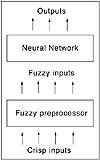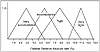# 20.C++ Neural Networks and Fuzzy Logic by Valluru B. Rao M&T Books, IDG Books Worldwide, Inc. ISBN: 1558515526   Pub Date: 06/01/95

### Fuzziness in Neural Networks

There are a number of ways fuzzy logic can be used with neural networks. Perhaps the simplest way is to use a fuzzifier function to preprocess or post-process data for a neural network. This is shown in Figure 3.1, where a neural network has a preprocessing fuzzifier that converts data into fuzzy data for application to a neural network.Figure 3.1  A neural network with fuzzy preprocessor.

Let us build a simple fuzzifier based on an application to predict the direction of the stock market. Suppose that you wish to fuzzify one set of data used in the network, the Federal Reserve’s fiscal policy, in one of four fuzzy categories: very accommodative, accommodative, tight, or very tight. Let us suppose that the raw data that we need to fuzzify is the discount rate and the interest rate that the Federal Reserve controls to set the fiscal policy. Now, a low discount rate usually indicates a loose fiscal policy, but this depends not only on the observer, but also on the political climate. There is a probability, for a given discount rate that you will find two people who offer different categories for the Fed fiscal policy. Hence, it is appropriate to fuzzify the data, so that the data we present to the neural network is like what an observer would see.

Figure 3.2 shows the fuzzy categories for different interest rates. Note that the category tight has the largest range. At any given interest rate level, you could have one possible category or several. If only one interest rate is present on the graph, this indicates that membership in that fuzzy set is 1.0. If you have three possible fuzzy sets, there is a requirement that membership add up to 1.0. For an interest rate of 8%, you have some chance of finding this in the tight category or the accommodative category. To find out the percentage probability from the graph, take the height of each curve at a given interest rate and normalize this to a one-unit length. At 8%, the tight category is about 0.8 unit in height, and accommodative is about 0.3 unit in height. The total is about 1.1 units, and the probability of the value being tight is then 0.8/1.1 = 0.73, while the probability of the value being accommodative is 0.27.Figure 3.2  Fuzzy categories for Federal Reserve policy based on the Fed discount rate.

### Code for the Fuzzifier

Let’s develop C++ code to create a simple fuzzifier. A class called category is defined in Listing 3.1. This class encapsulates the data that we need to define, the categories in Figure 3.2. There are three private data members called lowval, midval, and highval. These represent the values on the graph that define the category triangle. In the tight category, the lowval is 5.0, the midval is 8.5, and the highval is 12.0. The category class allows you to instantiate a category object and assign parameters to it to define it. Also, there is a string called name that identifies the category, e.g. “tight.” Various member functions are used to interface to the private data members. There is setval(), for example, which lets you set the value of the three parameters, while gethighval() returns the value of the parameter highval. The function getshare() returns the relative value of membership in a category given an input. In the example discussed earlier, with the number 8.0 as the Fed discount rate and the category tight defined according to the graph in Figure 3.2, getshare() would return 0.8. Note that this is not yet normalized. Following this example, the getshare() value from the accommodative category would also be used to determine the membership weights. These weights define a probability in a given category. A random number generator is used to define a value that is used to select a fuzzy category based on the probabilities defined.

Listing 3.1 fuzzfier.h

` // fuzzfier.h V. Rao, H. Rao // program to fuzzify data class category { private:      char name;      float   lowval,highval,midval; public:      category(){};      void setname(char *);      char * getname();      void setval(float&,float&,float&);      float getlowval();      float getmidval();      float gethighval();      float getshare(const float&);      ~category(){}; }; int randnum(int); `C++ Neural Networks and Fuzzy Logic
ISBN: 1558515526
EAN: 2147483647
Year: 1995
Pages: 139

Similar book on Amazon